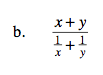Home > A2C > Chapter 7 > Lesson 7.2.3 > Problem7-131

7-131.
1. Simplify the following fractions. Homework Help ✎

1.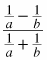2.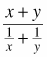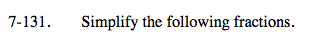Remove the fractions in the numerator and in the denominator. See problem 7-97 for help.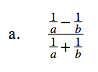$\frac{\textit{b}-\textit{a}}{\textit{b}+\textit{a}}$